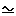# Maths - Spinors and Even Clifford Subalgebras

On this page we discussed some properties of Spinors and here we want to relate the following properties:

• They can represent conventional (360°) rotations in 'n' dimensions.
• They are represented by even Clifford subalgebras.
• They are related to spin rotations with 2:1 ratio of angles.

Ideally I would like to show that these properties are a consequence of each other.

I think they are all related because rotations in 'n' dimensions are equivalent to two reflections. I missed this until now because I thought that the equivalence of rotation and 2 reflections was just a curiosity, I still don't like it because I think of a rotation as a continuous thing which can be differentiated etc. Not a sequence of jumps! I still don't like this but I'm starting to think its more fundamental than first thought?

One reflection is given by:

p' = -n p n

where:

• p = vector being reflected
• n = unit vector which is normal to the mirror (plane in which p is reflected)

So it is similar to the rotation sandwich product except that, there is a negative sign, there is no inverse, and all quantities are vectors (odd grade).

Two reflections are given by applying this twice:

p' = n2 n1 p n1 n2

where:

• n2 n1 = unit bivector (even grade)
• n1 n2 = inverse (reverse) of unit bivector

So this is the rotation sandwich product.

We could keep repeating this to give trivectors, quadvectors... but only even grades will be free of reflections.

The 2:1 ratio of angles comes from the fact that the rotation is twice the angle between the mirrors.

## Reflection and Rotation of basis vectors

If we are working in 3D Euclidean space then:

1) the basis vectors are e1, e2 and e3
2) e1e1 = e2e2 = e3e3 = +1 (assuming space vectors square to +1 !)
3) ei ej = -ej ei (all vectors anti-commute)

Let n = e1 = normal to mirror (so mirror is in e2^e3 plane)

So how are the basis vectors transformed by the mirror?
e1' = -e1 e1 e1 = -e1
e2' = -e1 e2 e1 = +e2 e1 e1= +e2
e3' = -e1 e3 e1 = +e3 e1 e1= +e3

So as we would expect, components of vectors parallel to the mirror are unchanged, components of vectors perpendicular to the mirror are inverted.

So now lets reflect in e1 followed by e2, which should give a 180° rotation, how are the basis vectors transformed by the two mirrors?
e1' = e2 e1 e1 e1 e2 = -e1
e2' = e2 e1 e2 e1 e2 = -e2
e3' = e2 e1 e3 e1 e2 = +e3

Both e1 and e2 are inverted which is what we would expect if we rotate 180° around e3.

## Reflection and Rotation of linier sum

Now for the more general case, the normal to the first mirror is:
(a e1 + b e2 + c e3)
and the normal to the second mirror is:
(d e1 + e e2 + f e3)

so the spinor is:
(a e1 + b e2 + c e3)(d e1 + e e2 + f e3)
= a*d + b*e + c*f + (a*e - b*d)e1e2 + (b*f - c*e)e2e3+ (c*d - a*f)e3e1
= even Cliffordquaternion in 3D case

Where I can, I have put links to Amazon for books that are relevant to the subject, click on the appropriate country flag to get more details of the book or to buy it from them.Clifford Algebras and Spinors (London Mathematical Society Lecture Note S.)   Pertti Lounesto. This is very complex subject matter, however there is a lot of explanations and it is not all proofs like some mathematical textbooks. The book has a lot of information about the relationship between Clifford Algebras and Hypercomplex Algebras.

Other Math Books